• 基于FPGA灰度图像的形态学膨胀算法的实现1 背景知识腐蚀与膨胀是形态学滤波的两个基本运算，通过腐蚀和膨胀两种运算可以实现多种功能，主要如下：（1）消除噪声；（2）分割出独立的...

基于FPGA灰度图像的形态学膨胀算法的实现

1 背景知识

腐蚀与膨胀是形态学滤波的两个基本运算，通过腐蚀和膨胀两种运算可以实现多种功能，主要如下：

（1） 消除噪声；

（2）分割出独立的图像元素；

（3）在图像中连接相邻的元素；

（4）寻找图像中明显的极大值和极小值区域；

（5）求出图像的梯度。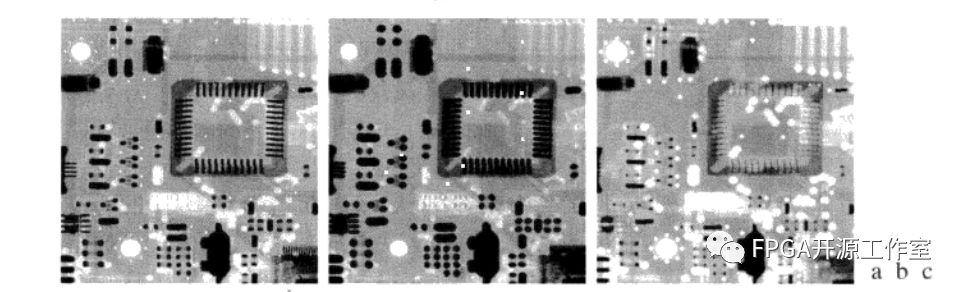图1 腐蚀膨胀示意图

图1 a为大小为448X425像素的灰度级X射线图像；b使用半径为2个像素的圆盘形结构元对图像的腐蚀结果；c用相同的结构元对图像的膨胀结果。原图有Lixi公司提供。

1）形态学滤波之膨胀

膨胀(dialate)就是求局部最大值的操作。

从数学角度来看就是将图像f和核（结构元）b进行卷积的一个过程。

当b的原点位于（x,y）处时，用一个平坦的结构元b在（x,y）处对图像f的膨胀，定义为图像f中与b重合区域的最大值，即：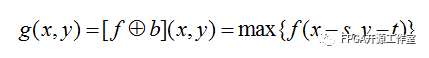为了方便起见，将膨胀操作记为：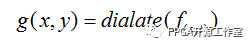(x,y)表示当前输入图像的行列坐标；

f(x,y)表示坐标点（x,y）处的图像像素值；

g(x,y)表示坐标点（x,y）处的滤波结果；

（s,t）表示作用域。

2 matlab仿真灰度图像的腐蚀与膨胀

Matlab膨胀源码：

%%image dilate

clc

clear all

figure,imshow(img_a);

title('img_a rgb');

img_b = rgb2gray(img_a);

figure,imshow(img_b);

title('img_b gary');

a = [1,1,1;

1,1,1;

1,1,1]; %structural element

b = [1,1,1,1,1;

1,1,1,1,1;

1,1,1,1,1;

1,1,1,1,1;

1,1,1,1,1];

c = [1,1,1,1,1,1,1;

1,1,1,1,1,1,1;

1,1,1,1,1,1,1;

1,1,1,1,1,1,1;

1,1,1,1,1,1,1;

1,1,1,1,1,1,1;

1,1,1,1,1,1,1];

img_c = imdilate(img_b,a);

figure,imshow(img_c);

title('img_c 3x3');

img_d = imdilate(img_b,b);

figure,imshow(img_d);

title('img_d 5x5');

img_e = imdilate(img_b,c);

figure,imshow(img_e);

title('img_e 7x7');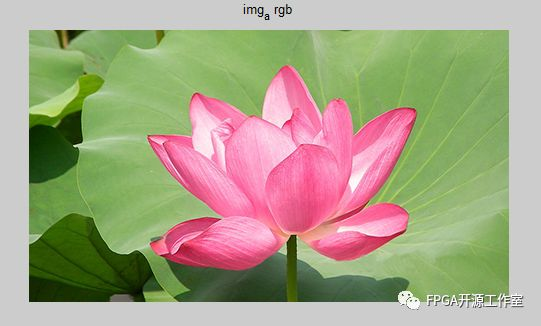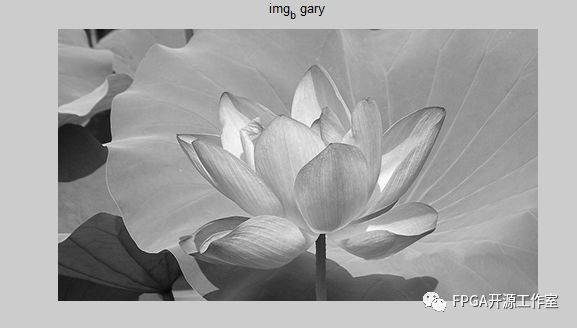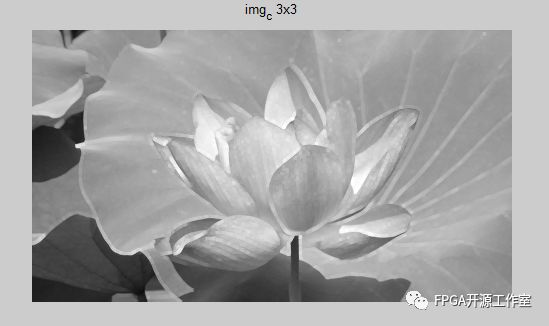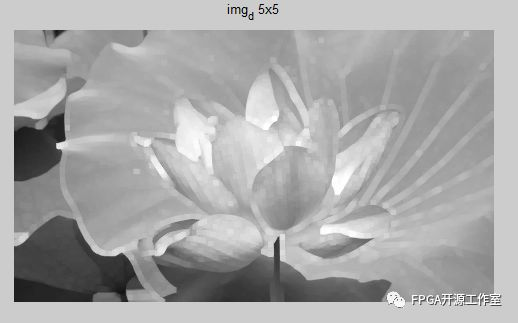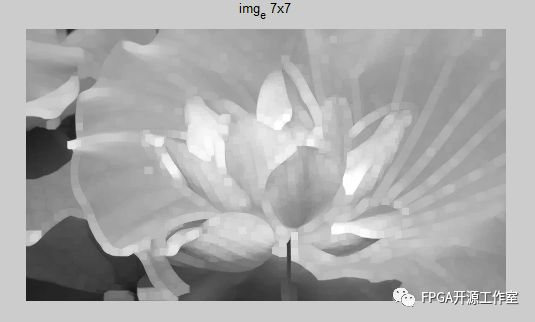3.1 膨胀模块的设计

1）比较子模块

2）一维形态学膨胀子模块

3）二维形态学腐蚀子模块

（1） 比较子模块

为了代码更好的移植，我们将比较子模块设计为独立的子模块。

Erode：输出俩个数据的较小值。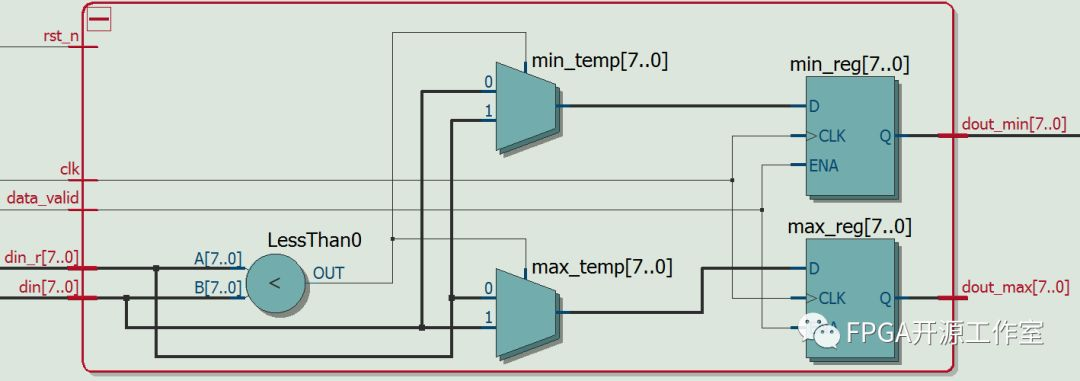比较子模块源码：

/*

Module name:  minmax.v

Description:

Data:         2018/03/14

Engineer:     lipu

e-mail:       137194782@qq.com

*/

timescale 1ns/1ps

module minmax(

clk,  //pixel clock

rst_n,

data_valid,

din,

din_r,

dout_min,

dout_max

);

parameter WIDTH = 8; // data width 8 bit

parameter USE_REG = 1; // USE_REG = 1 Data delay 1ns output.  USE_REG = 0 Don't delay.

input               clk;  //pixel clock

input               rst_n;

input               data_valid;

input  [WIDTH-1:0] din;

input  [WIDTH-1:0] din_r;

output [WIDTH-1:0] dout_min;

output [WIDTH-1:0] dout_max;

wire minmax_flag;

wire [WIDTH-1:0] min_temp;

wire [WIDTH-1:0] max_temp;

reg  [WIDTH-1:0] min_reg;

reg  [WIDTH-1:0] max_reg;

// min or max flag

assign minmax_flag = (din > din_r) ? 1'b1:1'b0;

// min

assign min_temp = (minmax_flag == 1'b1) ? din_r : din;

// max

assign max_temp = (minmax_flag == 1'b1) ? din : din_r;

// USE_REG == 1

generate

if(USE_REG == 1)

begin : MAP0

always @(posedge clk) begin

if(data_valid) begin

min_reg <= #1 min_temp;

max_reg <= #1 max_temp;

end

end

assign dout_min = min_reg;

assign dout_max = max_reg;

end

endgenerate

//USE_REG == 0

generate

if(~(USE_REG == 1))

begin : MAP1

assign dout_min = min_temp;

assign dout_max = max_temp;

end

endgenerate

endmodule

比较子模块仿真源码：

/*

Module name:  minmax_tb.v

Description:

*/

timescale 1ns/1ps

define WIDTH 8

define CLK_PERIOD 10

module minmax_tb();

reg               clk;  //pixel clock

reg               rst_n;

reg               data_valid;

reg  [WIDTH-1:0] din;

reg  [WIDTH-1:0] din_r;

wire [WIDTH-1:0] dout_min;

wire [WIDTH-1:0] dout_max;

minmax minmax_inst(

.clk(clk),  //pixel clock

.rst_n(rst_n),

.data_valid(data_valid),

.din(din),

.din_r(din_r),

.dout_min(dout_min),

.dout_max(dout_max)

);

initial begin

clk = 0;

rst_n = 0;

data_valid = 0;

#(CLK_PERIOD*10);

rst_n = 1;

#(CLK_PERIOD*10);

data_valid = 1;

#(CLK_PERIOD*100);

data_valid = 0;

#(CLK_PERIOD*10);

$stop; end always #(CLK_PERIOD/2)clk = ~clk; always @(posedge clk or negedge rst_n) begin if(!rst_n) din <= 8'd0; else if(data_valid) din <= {$random}%255;

else

din <= 8'b0;

end

always @(posedge clk or negedge rst_n) begin

if(!rst_n)

din_r <= 8'd0;

else if(data_valid)

din_r <= din;

else

din_r <= 8'b0;

end

Endmodule

比较子模块仿真波形：

t

t+1

t+2

t+3

t+4

t+5

t+6

t+7

t+8

t+9

t+10

din

128

42

232

92

72

77

95

37

216

184

198

din_r

X

128

42

232

92

72

77

95

37

216

184

dout_min

X

X

42

42

92

72

72

77

37

37

184

dout_max

X

X

128

232

232

92

77

95

95

216

216

当我们需要做膨胀算法时，数据取dout_max;当我们需要做腐蚀算法时，数据取dout_min。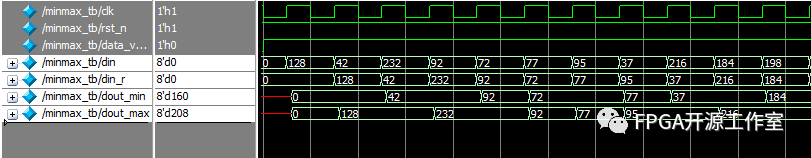（2）一维形态学腐蚀膨胀模块设计

我们要完成对nxn窗口的腐蚀或者膨胀首先我们要做图像行的一维腐蚀或膨胀。例如我们要做3x3窗口的腐蚀或膨胀，一维形态学腐蚀或膨胀如图所示：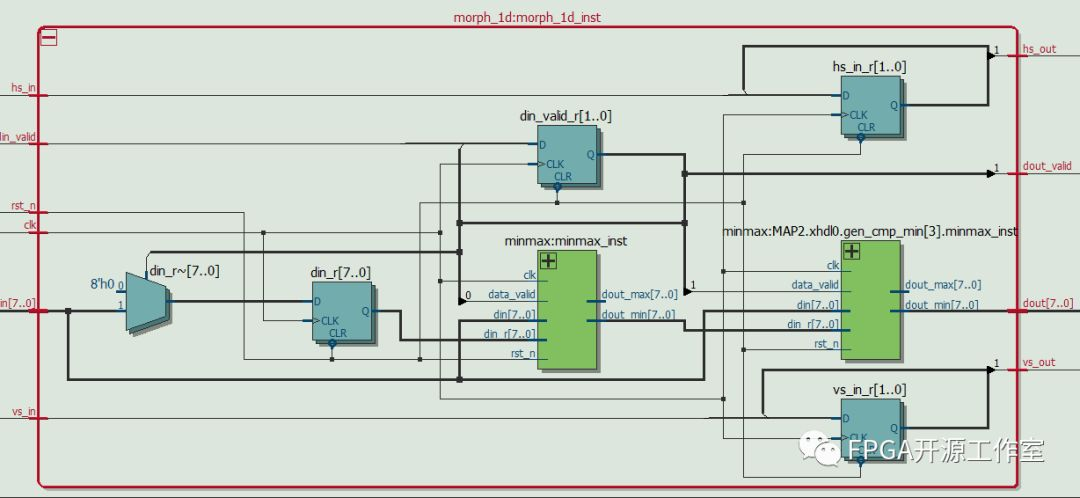(3) 二维形态学腐蚀与膨胀子模块设计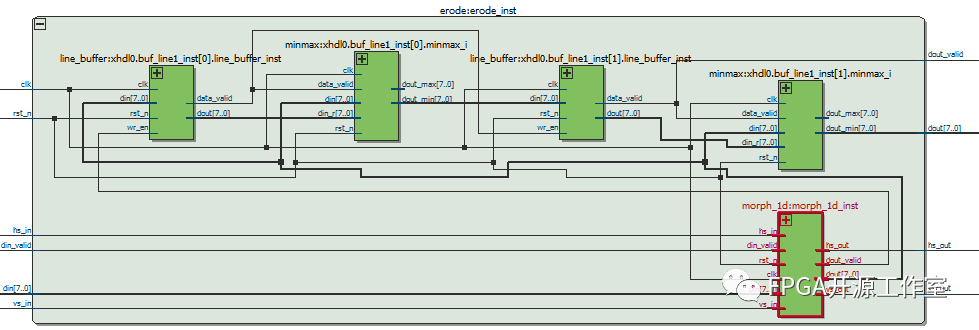形态学膨胀结果演示：

顶层源码：

wire [15:0] rgb;

wire hs;

wire vs;

wire de;

wire o_hs;

wire o_vs;

wire o_de;

wire [7 : 0]o_y_8b;

wire [7 : 0]o_cb_8b;

wire [7 : 0]o_cr_8b;

wire [7 : 0]      dout;

//assign TFT_rgb = {o_y_8b[7:3],o_y_8b[7:2],o_y_8b[7:3]};     //Y

//assign TFT_rgb = {o_cb_8b[7:3],o_cb_8b[7:2],o_cb_8b[7:3]};  //cb

//assign TFT_rgb = {o_cr_8b[7:3],o_cr_8b[7:2],o_cr_8b[7:3]};  //cr

tft_ctrl tft_ctrl(

.Clk9M(clk9M),//系统输入时钟9MHZ

.Rst_n(Rst_n),//复位输入，低电平复位

.data_in({Rd_data[7:0],Rd_data[15:8]}),//待显示数据

.hcount(),//TFT行扫描计数器

.vcount(),//TFT场扫描计数器

.TFT_RGB(rgb),//TFT数据输出

.TFT_HS(hs),//TFT行同步信号

.TFT_VS(vs),//TFT场同步信号

.TFT_CLK(TFT_clk),//TFT像素时钟

.TFT_DE(de),//TFT数据使能

.TFT_begin(tft_begin),

.TFT_PWM(TFT_pwm)//TFT背光控制

);

rgb_to_ycbcr  rgb_to_ycbcr_inst(

.clk(TFT_clk),

.i_r_8b({rgb[15:11],3'b0}),

.i_g_8b({rgb[10:5],2'b0}),

.i_b_8b({rgb[4:0],3'b0}),

.i_h_sync(hs),

.i_v_sync(vs),

.i_data_en(de),

.o_y_8b(o_y_8b),

.o_cb_8b(o_cb_8b),

.o_cr_8b(o_cr_8b),

.o_h_sync(o_hs),

.o_v_sync(o_vs),

.o_data_en(o_de)

);

/*

erode erode_inst(

.clk(TFT_clk),

.rst_n(Rst_n),

.hs_in(o_hs),

.vs_in(o_vs),

.din(o_y_8b),

.din_valid(o_de),

.dout(dout),

.dout_valid(TFT_de),

.hs_out(TFT_hs),

.vs_out(TFT_vs)

);

*/

dilate dilate_inst(

.clk(TFT_clk),

.rst_n(Rst_n),

.hs_in(o_hs),

.vs_in(o_vs),

.din(o_y_8b),

.din_valid(o_de),

.dout(dout),

.dout_valid(TFT_de),

.hs_out(TFT_hs),

.vs_out(TFT_vs)

);

assign TFT_rgb = {dout[7:3],dout[7:2],dout[7:3]};     //Y

//assign TFT_rgb = {o_y_8b[7:3],o_y_8b[7:2],o_y_8b[7:3]};     //Y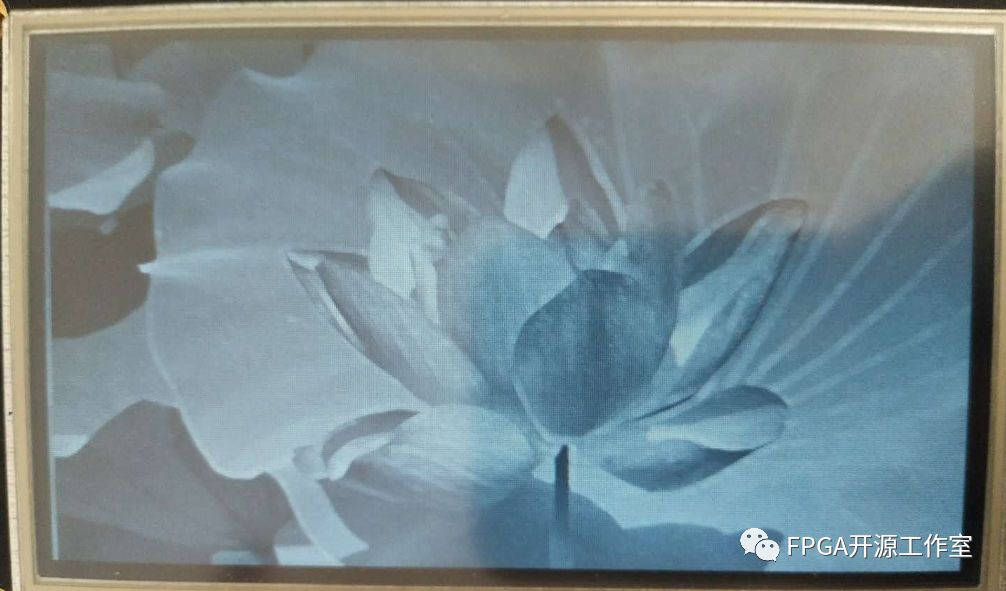展开全文FPGA
• Delphi源码，演示了数字图像处理中的形态学算法的膨胀算法。 本演示使用形态学膨胀算法来弥补裂纹。
• 数字图像处理形态学算法中膨胀算法基于MATLAB实现（自己编写），不是调用库函数
• MATLAB三维形态学腐蚀膨胀操作代码。做三维形态学操作的时候，没找到MATLAB自带的函数，所以自己写了一个。
（MATLAB）三维形态学腐蚀膨胀算法（代码）膨胀腐蚀结构元与其他函数测试其他
by HPC_ZY
MATLAB三维形态学腐蚀膨胀操作代码。做三维形态学操作的时候，没找到MATLAB自带的函数，所以自己写了一个。
膨胀
膨胀比较简单，总之就是原始图像某一位置为1时，把周围的全置为1
%% 三维膨胀
function out = dilate3d(in,r)

[R,C,S] = size(in);
% 生成球形结构元
[se,n,rx,ry,rz] = strel3d(r);

out = false(R,C,S);
for i = 1+rx:R-rx
for j = 1+ry:C-ry
for k = 1+rz:S-rz
if in(i,j,k) == 1
for idx = 1:n
out(i+se(idx,1),j+se(idx,2),k+se(idx,3)) = 1;
end
end
end
end
end

end


腐蚀
与膨胀相反，当原始图像某邻域范围内全为1，中心点才能置为1
%% 三维腐蚀
function out = erode3d(in,r)
% 生成球形结构元
[R,C,S] = size(in);
[se,n,rx,ry,rz] = strel3d(r);

out = false(R,C,S);
for i = 1+rx:R-rx
for j = 1+ry:C-ry
for k = 1+rz:S-rz
out(i,j,k) = 1;
for idx = 1:n
if in(i+se(idx,1),j+se(idx,2),k+se(idx,3))<1
out(i,j,k) = 0;
break;
end
end
end
end
end

end


结构元与其他函数
生成结构元的函数，建议三个方向半径一致。
注：由于此处结构元特殊的判定方式，当三个方向半径不一致时，最终尺寸不一定等于用户输入的尺寸，但大体形态是相同的
%% 生成三维球形结构元
function [se,n,rx,ry,rz] = strel3d(r)

% 判断用户输入半径
if length(r)<2
rx = r;
ry = r;
rz = r;
elseif length(r)>2
rx = r(1);
ry = r(2);
rz = r(3);
else
rx = r(1);
ry = r(1);
rz = r(2);
end

% 生成球形二值图 并获得目标索引
[x,y,z] = meshgrid(-rx:rx,-ry:ry,-rz:rz);
[x,y,z] = find3d(sqrt(x.^2+y.^2+z.^2)<(rx+ry+rz)/2.5); % 注意这里的判定方式
se = [x-rx-1,y-ry-1,z-rz-1];

n = size(se,1);

end

find3函数（类似MATLAB自带的find，但这里是三维的）
%% 找到满足条件的值，类似MATLAB自带的find，但这里是三维的
function [x,y,z] = find3d(in)

[M,N,~] = size(in);

idx = find(in);

z = ceil(idx/M/N);
idx = idx-(z-1)*M*N;
y = ceil(idx/M);
x = idx-(y-1)*M;

end


测试
clear; close all; clc

%% 生成测试图像（三维）
im = zeros(50,50,50);
im(20:30,20:30,10:40) = 1;

%% 形态学操作
% 膨胀
im1 = dilate3d(im,5);

% 腐蚀
im2 = erode3d(im,3);
im3 = erode3d(im,[3,3,10]);

%% 显示结果
viewer3d(im,double(im1),im,double(im2),im,double(im3))
% 注意，仅仅运行到这里，是什么都不会显示的哦
% viewer3d是可视化界面，你得和它互动，要显示什么你的自己学。
% 这个东西怎么用，就参考“其他=》2.关于viewer3d函数”

测试结果如下，其中 红色为原始模型，绿色为操作后模型，黄色为重叠部分其他

为了简便易理解，所以没有进行优化，运行速度一般
关于viewer3d函数，可查看另一篇文章
三维开闭运算可以组合腐蚀膨胀实现，这里就不贴代码了
如果有其他问题或需求，欢迎评论区留言或私信


展开全文MATLAB
• 腐蚀：是膨胀的反操作，一般来说膨胀会扩张区域和腐蚀缩小区域膨胀可以填补细小的坑洞而腐蚀可以消除细的突起，maltab中使用imdalite(A,B)表示使用核B膨胀A区域，核B可以通过strel函数获得，如strel('disk',3)表示...
膨胀：是将A与核B卷积，求局部最大值，可以使得图像中的亮区域逐渐增长；腐蚀：是膨胀的反操作，一般来说膨胀会扩张区域和腐蚀缩小区域膨胀可以填补细小的坑洞而腐蚀可以消除细的突起，maltab中使用imdalite(A,B)表示使用核B膨胀A区域，核B可以通过strel函数获得，如strel('disk',3)表示半径为3的圆。maltab中使用imerode(A,B),核B可以通过strel函数获得，如strel('disk',3)表示半径为3的圆。A  = imread('target.png');B = strel('disk',10);A1 = imdilate(A,B);A2 = imerode(A,B);A3 = imdilate(A,1B);subplot(221),imshow(A1;title('imdilate膨胀原始图像');subplot(222),imshow(A1);title('使用B后1次膨胀后的图像');subplot(223),imshow(A2);title('使用B后2次膨胀后的图像');subplot(224),imshow(A3);title('使用B后3次膨胀后的图像');如上图可见膨胀可以把白色区域放大，把细小的黑色区域填满(填满的大小取决于B核)，腐蚀则是黑色区域填充白色区域，白色细小区域被填满(原图来源冈萨雷斯书)。.开运算和闭运算都包含膨胀与腐蚀操作，开运算是先腐蚀在膨胀，其作用是消除小的亮孤立点，剩余两区域基本不变；闭运算是先膨胀再腐蚀，起作用是将亮区域连接在一起，迭代开运算或者是闭运算不是膨胀--腐蚀--膨胀--腐蚀而是膨胀--膨胀--腐蚀--腐蚀(这个是在opencv中会用到)。a = imread('target.png');B=strel('disk',10);A1=imopen(a,B);A2=imclose(a,B);A3 = imclose(A1,B);A4 = imopen(A2,B);subplot(221),imshow(A1);title('开运算图像');subplot(222),imshow(A2);title('闭运算图像');subplot(223),imshow(A3);title('先开后闭图像');subplot(224),imshow(A4);title('先闭后开图像');通过上图可以看出开运算把孤立的白色区域覆盖了，而闭运算把黑色区域覆盖了，将两者结合，可以消除孤立点可用于除噪。当图像相减与开运算和闭运算结合的时候会产生顶帽变换和底帽变换，顶帽是图像减去开运算操作，而底帽是图像减去闭运算操作； 顶帽运算常用于暗背景上的亮物体，而底帽变换则用于相反的情况，在opencv中底帽变换被称为黑帽就是这个原因(我猜的因为黑底帽变换，省去了底字，但是maltab省去了黑字)。对于这两个运算针对不同的图片会有不同的想象，可以多试下，我随便找个图像试的，相比之前多了一些文理，也算是增加了对比度吧，这两个在分割这一块用的比较多。mark(2015 12 13)
展开全文• 使用两种大小尺寸的形态学膨胀算子优化带内重要系数编码，并对两种膨胀方式采用不同的算术编码模型，克服了传统单一算子不能平衡膨胀速度和膨胀质量的不足．使用差分缩减方式对各小波系数聚类簇的起始 位置和稀疏...
• 数学形态学腐蚀膨胀运算的快速算法。介绍了形态学的两种运算：腐蚀和膨胀的快速预算方法。
• 图像的形态学处理，可以完成开运算 闭运算 腐蚀 膨胀 TopHat变换，形态学梯度处理等算法，对于学习图像处理有很大的帮助
• 形态学——腐蚀,膨胀,细化算法，用的c语言实现，可以参考一下
• 膨胀算法4.其它相关图像处理函数RGB图像转灰度图阈值法灰度图转二值图开运算闭运算三、主函数调用处理结果 前言 虽然大部分情况下，我们直接调用cv库就可以完成膨胀腐蚀的操作，但如果在“完成老师的任务”的情况下...
用Python实现形态学处理（膨胀、腐蚀算法）
在不调用opencv库的情况下，利用卷积的思路实现膨胀腐蚀算法

文章目录用Python实现形态学处理（膨胀、腐蚀算法）前言一、膨胀与腐蚀的简介腐蚀(erode)膨胀(dilate)二、针对结构元素不规则的情况1.调用库函数2.腐蚀算法3.膨胀算法4.其它相关图像处理函数RGB图像转灰度图阈值法灰度图转二值图开运算闭运算三、主函数调用处理结果

前言
虽然大部分情况下，我们直接调用cv库就可以完成膨胀腐蚀的操作，但如果在“完成老师的任务”的情况下，自己想办法做一下膨胀腐蚀运算还是有必要的。
与常见的“扫描-判定”的算法不同，本文提出了一种利用卷积的思路，实现适应自定义非规则的结构元素及中心点进行膨胀腐蚀运算的方法。

提示：以下是本篇文章正文内容，下面案例可供参考
一、膨胀与腐蚀的简介
简单介绍二值图像、膨胀、腐蚀的概念
图像像素中只有0和1的图像称为二值图像，其中0区域显示为黑色，1区域显示为白色膨胀与腐蚀，都是通过结构元素在二值图像上做遍历的运算。
接下来以实例说明该种运算——
现有一目标图像，其中像素值只有1和0，结构元素为3×3，原点在正中心，如图所示：腐蚀(erode)
腐蚀可以理解为一种“与”运算，在结构元素在目标图像上移动的过程中，若结构元素内的像素值全为1（即结构元素与目标区域完全“重合”）时，将结构元素的原点位置的像素值设置为1。
遍历完毕后，将其它位置的像素值均设为0。
腐蚀结果如下：膨胀(dilate)
膨胀预算可以理解为一种“或”运算，在结构元素在目标图像上移动的过程中，若结构元素内存在像素值为1（即结构元素与目标区域存在“重合”部分）时，将结构元素的原点位置的像素设置为1。
遍历完毕后，将其它位置的像素值均设为0。
膨胀结果如下：二、针对结构元素不规则的情况
针对规则的结构元素，我们可以采用“搜索-验证”的逻辑进行处理，即找到目标图像中每次结构元素移动所对应的规则小矩形，对其所有像素值进行判定，对其中心原点进行标记。
但进一步的，假设，在某种情况下，我希望程序能够实现使用不规则的结构元素对目标图像进行膨胀与腐蚀操作，该如何实现？
这里我引入一个卷积（自相关）的思路：
现在，假设我们目标图像不变，结构元素与其原点均不规则，如图：它的腐蚀、膨胀结果将如下：（有兴趣可以自己手工做一些有所体会）假设结构元素中，属于其的部分为1，不属于结构元素的部分为0。
可以验证如下事实：
当结构元素与目标图像中与其对应的小像素矩阵的卷积和与其“面积”（非0像素点个数之和）相等时，说明发生了“重合”，中心点位置像素值设为1，不等时为0。
当结构元素与目标图像中与其对应的小像素矩阵的卷积和大于0时，说明有重合部分，中心点像素值设为1，卷积和等于0时设为0。
核心代码如下：
1.调用库函数
可以看到，引入的库文件中不包括cv库：
import numpy as np
from matplotlib import pyplot as plt
from PIL import Image
import matplotlib as mpl

2.腐蚀算法
输入有三个变量，分别为待处理的二值图像bin_im，结构元素kernel，结构元素的原点位置（起始点为[0,0]）：
def img_erode(bin_im, kernel, center_coo):
kernel_w = kernel.shape
kernel_h = kernel.shape
if kernel[center_coo, center_coo] == 0:
raise ValueError("指定原点不在结构元素内！")
erode_img = np.zeros(shape=bin_im.shape)
for i in range(center_coo, bin_im.shape-kernel_w+center_coo+1):
for j in range(center_coo, bin_im.shape-kernel_h+center_coo+1):
a = bin_im[i-center_coo:i-center_coo+kernel_w,
j-center_coo:j-center_coo+kernel_h]  # 找到每次迭代中对应的目标图像小矩阵
if np.sum(a * kernel) == np.sum(kernel):  # 判定是否“完全重合”
erode_img[i, j] = 1
return erode_img

3.膨胀算法
输入有三个变量，分别为待处理的二值图像bin_im，结构元素kernel，结构元素的原点位置（起始点为[0,0]）。
def img_dilate(bin_im, kernel, center_coo):
kernel_w = kernel.shape
kernel_h = kernel.shape
if kernel[center_coo, center_coo] == 0:
raise ValueError("指定原点不在结构元素内！")
dilate_img = np.zeros(shape=bin_im.shape)
for i in range(center_coo, bin_im.shape - kernel_w + center_coo + 1):
for j in range(center_coo, bin_im.shape - kernel_h + center_coo + 1):
a = bin_im[i - center_coo:i - center_coo + kernel_w,
j - center_coo:j - center_coo + kernel_h]
dilate_img[i, j] = np.max(a * kernel)  # 若“有重合”，则点乘后最大值为0
return dilate_img

4.其它相关图像处理函数
RGB图像转灰度图
def rgb2gray(rgb_img):
gray = rgb_img[:, :, 0] * 0.299 + rgb_img[:, :, 1] * 0.587 + rgb_img[:, :, 2] * 0.114
return gray

阈值法灰度图转二值图
def im_binary(gray_image, t=80):
binary_image = np.zeros(shape=(gray_image.shape, gray_image.shape), dtype=np.uint8)
for i in range(gray_image.shape):
for j in range(gray_image.shape):
if gray_image[i][j] > t:
binary_image[i][j] = 1
else:
binary_image[i][j] = 0
return binary_image

开运算
先腐蚀再膨胀
def img_open(bin_im, erope_k, erope_c_c, dilate_k, dilate_c_c):
open_img = img_erode(bin_im, erope_k, erope_c_c)
open_img = img_dilate(open_img, dilate_k, dilate_c_c)
return open_img

闭运算
先膨胀再腐蚀
def img_close(bin_im, erope_k, erope_c_c, dilate_k, dilate_c_c):
close_img = img_dilate(bin_im, dilate_k, dilate_c_c)
close_img = img_erode(close_img, erope_k, erope_c_c)
return close_img

三、主函数调用
if __name__ == "__main__":
img = np.array(Image.open(r".\logo.jpg"))  # 此处可以是指定路径的rgb图像
gray_img = 255 - rgb2gray(img)
print("原始二值图像")
bin_img = im_binary(gray_img)
kernel1 = np.ones(shape=(3, 3))  # 此处声明结构元素，可以自定义为非规则形状
center1 = [1, 1]  # 此处声明结构元素原点，这里取其正中心像素点
kernel2 = np.ones(shape=(5, 5))  # 同上
center2 = [2, 2]  # 同上
print("原始二值图像腐蚀")
erode_im = img_erode(bin_img, kernel2, center2)
print("原始二值图像膨胀")
dilate_im = img_dilate(bin_img, kernel2, center2)
print("原始二值图像开运算")
open_im = img_open(bin_img, kernel1, center1, kernel1, center1)
print("原始二值图像闭运算")
close_im = img_close(bin_img, kernel1, center1, kernel1, center1)

plt.subplot(241)
plt.title("原始图像")
plt.imshow(img)
plt.subplot(242)
plt.title("灰度图像")
plt.imshow(gray_img, cmap="gray")
plt.subplot(243)
plt.title("二值图像")
plt.title("二值图像")
plt.imshow(bin_img, cmap="gray")
plt.subplot(245)
plt.title("腐蚀运算")
plt.imshow(erode_im, cmap="gray")
plt.subplot(246)
plt.title("膨胀运算")
plt.imshow(dilate_im, cmap="gray")
plt.subplot(247)
plt.title("开运算")
plt.imshow(open_im, cmap="gray")
plt.subplot(248)
plt.title("闭运算")
plt.imshow(close_im, cmap="gray")
plt.show()


处理结果希望能够对做相关课后作业的其它同学有帮助。

`
展开全文python 卷积 矩阵
• 摘 要: 本文介绍了数学形态学中结构元素为4连通或8连通的3×3邻域时腐蚀、膨胀运算的快速算法。区域采用线段编码表示，腐蚀与膨胀运算在当前线段与其相邻的上下线段之间通过逻辑运算实现。4连通邻域结构元素下作腐蚀...
• 形态学常用的有腐蚀，膨胀，开运算，闭运算，顶帽  膨胀：是将A与核B卷积，求局部最大值，可以使得图像中的亮区域逐渐增长；  腐蚀：是膨胀的反操作，一般来说膨胀会扩张区域和腐蚀缩小区域  膨胀可以填补细小的...matlab 学习笔记
• 4形态学膨胀之连通域提取 提取连通域实际上是标记连通域的过程，其算法如下： 初始点：Bo=某个连通分量中的某点（这里通过遍历图像，知道像素值为255，将此点作为种子点） 循环： （用3X3d的结构元素对种子点...
• 若该文为原创文章，未经允许不得转载 原博主博客地址：...本文章博客地址： OpenCV开发笔记（二十一）：算法基础之形态学滤波-膨胀 前言 本篇章开始学习形态学滤波-膨胀。 ...图像处理
• 改进的膨胀算法在红外图像弱小目标检测中的应用，谷稳稳，刘建国，本文针对红外序列图像中弱小目标的检测问题，提出一种基于数学形态学膨胀算法的小目标检测方法。首先，利用弱小目标区域的不连续模式识别与智能系统
• 基本形态学算法 为什么要做基本形态学算法的研究和实现？是因为形态学是一个非常有力，应用 广泛的工具，但同时也是研究不是很清楚的工具。往往一个恰到好处的变换，就能够省下许多的劳动。对此的分类和研究就显得...
• 一、背景介绍 基于二值图像的滤波算法形态学滤波，在图像目标采集的预处理中经常被使用到，针对不同的使用场景涉及到腐蚀、膨胀、开闭运算等处理。实际使用中对于不同的分辨率大小以及模板窗...python java 计算机视觉 机器学习
• 本章介绍由数学形态学衍生的二值图像形态学算法，主要包括形态学膨胀、腐蚀、开运算和闭运算四种常用算法，并以此为基础讲解形态学轮廓提取算法，结合C语言编程实现，通俗易懂，图文并茂。
• 二值图像腐蚀操作属于图像形态学的范畴，形态学运算是只针对二值图像进行，并依据数学形态学(Mathermatical Morphogy)集合论方法发展起来的数字图像处理方法，它主要包括腐蚀，膨胀，开，闭，击中，击不中等。......